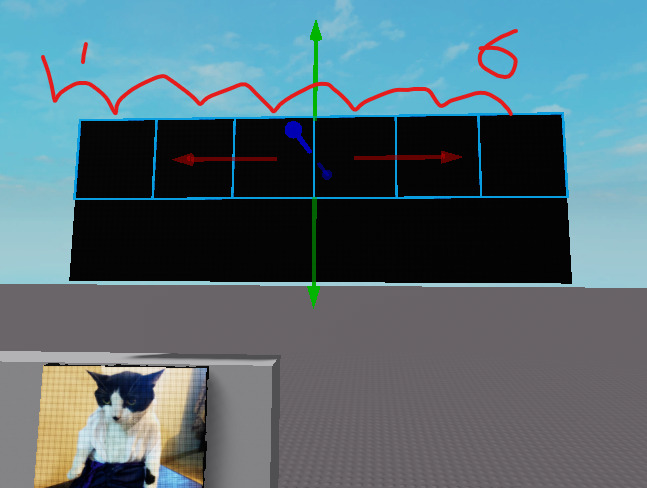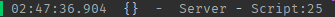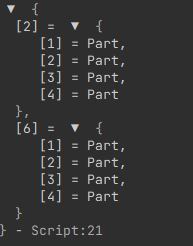# 4 by 4 Part Math

So, I’m trying to create a screen adorner. So I have 6 screens and they’re all 4 by 4 size. I’m trying to get the relative position. So I use

``````print(workspace.NScreens.S1.actualscreen.CFrame:ToObjectSpace(workspace.NScreens.S6.actualscreen.CFrame).Position)```
Which would say "20, 0, 0".
I need the script to know on how many screens there on the 'x-axis'.
So there should say that there are 6 screens on the x-axis. I don't know if this makes sense since its 1 am and I'm extremely tired.

Heres the image that didnt showYou could loop the model then get their y axis then store it on a table:

``````local tbl: { [yAxis]: { BasePart } } = {}

local model = script.Parent

for _, object: BasePart in ipairs(model:GetChildren()) do
if not object:IsA("BasePart") then
continue
end

local yPosition = math.floor(object.Position.Y)
local yAxisTable = tbl[yPosition]

if yAxisTable == nil then
tbl[yPosition] = {}
yAxisTable = tbl[yPosition]
end

table.insert(yAxisTable, object)
end
``````

I’m not sure if this worked or not since it doesn’t print anything out. This is my script and my way on getting relative position.

``````xtable={}
ytable={}
for i,v in pairs(workspace.NScreens:GetChildren()) do
--Get relative position from the first screen
local xval = workspace.NScreens.S1.actualscreen.CFrame:ToObjectSpace(v.actualscreen.CFrame).Position.X
local yval = workspace.NScreens.S1.actualscreen.CFrame:ToObjectSpace(v.actualscreen.CFrame).Position.Y

local positive=math.abs(yval)
table.insert(xtable, xval)
table.insert(ytable, positive)
end
-- Get the highest number in the table
local highestx = math.max(table.unpack(xtable))
local highesty = math.max(table.unpack(ytable))

print(highestx)
print(highesty)

for i,v in pairs(workspace.NScreens:GetChildren()) do
--Start putting frames into the screen
--This part doesn't excatly work.
wait (0.01)
print(v.Name)
local fframe = Instance.new("Frame")
fframe.Parent = v.actualscreen.SurfaceGui

local xlol = workspace.NScreens.S1.actualscreen.CFrame:ToObjectSpace(v.actualscreen.CFrame).Position.X
local ylol = workspace.NScreens.S1.actualscreen.CFrame:ToObjectSpace(v.actualscreen.CFrame).Position.Y
print("X = "..math.round(xlol))
print("Y = "..math.round(ylol))
fframe.Size = UDim2.new(xlol/4, 0, ylol/4, 0)
fframe.Position = UDim2.new(-xlol/4, 0, ylol/4, 0)
end
``````

What kind of statement is that? You do know you can just print it yourself, right? It’s probably time for you to sleep if you can’t even process this.

``````print(tbl)
``````I know how to print stuff. It’s really easy. I knew what to print.
What a surprise. Nothing happened! That’s what I meant when it doesn’t print anything outits a folder full of models anyway so it wont work

Of course it wont work because that is just an example and you have to modify it yourself.

``````local tbl: { [yAxis]: { BasePart } } = {}

local model = script.Parent

for _, object: BasePart in ipairs(model:GetChildren()) do
if not object:IsA("Model") then
continue
end

local screen: BasePart = object:FindFirstChild("Screen")

if not screen then
continue
end

local yPosition = math.floor(screen.Position.Y)
local yAxisTable = tbl[yPosition]

if yAxisTable == nil then
tbl[yPosition] = {}
yAxisTable = tbl[yPosition]
end

table.insert(yAxisTable, object)
end
``````